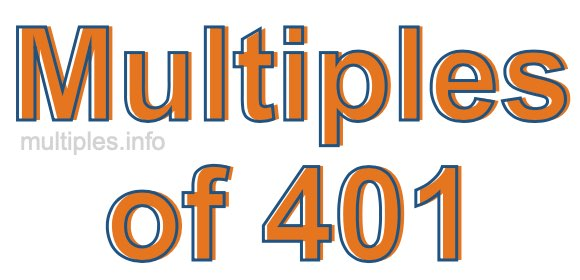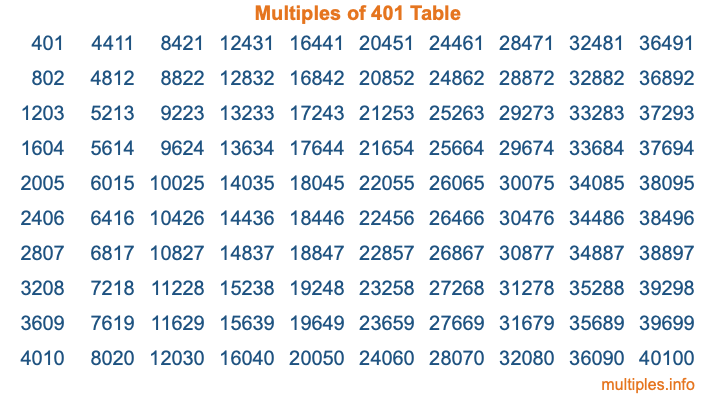Multiples of 401Welcome to the Multiples of 401 page. Here we will first teach you everything you will ever need to know about the multiples of 401, and then give you a study guide summary of everything we taught you to make sure you remember it all. Use this page to look up facts and learn information about the multiples of 401. This page will make you a multiples of four hundred one expert!

Definition of Multiples of 401
Multiples of 401 are all the numbers that when divided by 401 equal an integer. Each of the multiples of 401 are called a multiple. A multiple of 401 is created by multiplying 401 by an integer.

Therefore, to create a list of multiples of 401, you start with 1 multiplied by 401, then 2 multiplied by 401, then 3 multiplied by 401, and so on for as long as you want. Thus, the list of the first five multiples of 401 is 401, 802, 1203, 1604, and 2005. To see a larger list of multiples of 401, see the printable image of Multiples of 401 further down on this page. We also have a category where you can choose any nth multiple of 401.

Multiples of 401 Checker
The Multiples of 401 Checker below checks to see if any number of your choice is a multiple of 401. In other words, it checks to see if there is any number (integer) that when multiplied by 401 will equal your number. To do that, we divide your number by 401. If the the quotient is an integer, then your number is a multiple of 401.

Is  a multiple of 401?

Least Common Multiple of 401 and ...
A Least Common Multiple (LCM) is the lowest multiple that two or more numbers have in common. This is also called the smallest common multiple or lowest common multiple and is useful to know when you are adding our subtracting fractions. Enter one or more numbers below (401 is already entered) to find the LCM.

Check out our LCM Calculator if you need more details about the Least Common Multiple or if you need the LCM for different numbers for adding and subtraction fractions.

nth Multiple of 401
As we stated above, 401 is the first multiple of 401, 802 is the second multiple of 401, 1203 is the third multiple of 401, and so on. Enter a number below to find the nth multiple of 401.

th multiple of 401

Multiples of 401 vs Factors of 401
401 is a multiple of 401 and a factor of 401, but that is where the similarities end. All postive multiples of 401 are 401 or greater than 401. All positive factors of 401 are 401 or less than 401.

Below is the beginning list of multiples of 401 and the factors of 401 so you can compare:

Multiples of 401: 401, 802, 1203, 1604, 2005, etc.

Factors of 401: 1, 401

As you can see, the multiples of 401 are all the numbers that you can divide by 401 to get a whole number. The factors of 401, on the other hand, are all the whole numbers that you can multiply by another whole number to get 401.

It's also interesting to note that if a number (x) is a factor of 401, then 401 will also be a multiple of that number (x).

Multiples of 401 vs Divisors of 401
The divisors of 401 are all the integers that 401 can be divided by evenly. Below is a list of the divisors of 401.

Divisors of 401: 1, 401

The interesting thing to note here is that if you take any multiple of 401 and divide it by a divisor of 401, you will see that the quotient is an integer.

Multiples of 401 Table
Below is an image of the first 100 multiples of 401 in a table. The table is in chronological order, column by column. The first column has the first ten multiples of 401, the second column has the next ten multiples of 401, and so on.The Multiples of 401 Table is also referred to as the 401 Times Table or Times Table of 401. You are welcome to print out our table for your studies.

Negative Multiples of 401
Although not often discussed or needed in math, it is worth mentioning that you can make a list of negative multiples of 401 by multiplying 401 by -1, then by -2, then by -3, and so on, to get the following list of negative multiples of 401:

-401, -802, -1203, -1604, -2005, etc.

Multiples of 401 Summary
Below is a summary of important Multiples of 401 facts that we have discussed on this page. To retain the knowledge on this page, we recommend that you read through the summary and explain to yourself or a study partner why they hold true.

There are an infinite number of multiples of 401.

A multiple of 401 divided by 401 will equal a whole number.

401 divided by a factor of 401 equals a divisor of 401.

The nth multiple of 401 is n times 401.

The largest factor of 401 is equal to the first positive multiple of 401.

401 is a multiple of every factor of 401.

401 is a multiple of 401.

A multiple of 401 divided by a divisor of 401 equals an integer.

401 divided by a divisor of 401 equals a factor of 401.

Any integer times 401 will equal a multiple of 401.

Multiples of a Number
Here you can get the multiples of another number, all with the same attention to detail as we did for multiples of 401 on this page.

Multiples of
Multiples of 402
Did you find our page about multiples of four hundred one educational? Do you want more knowledge? Check out the multiples of the next number on our list!